# Solving multistep equationsPage 1

#### WATCH ALL SLIDES

Slide 1March 1, 2006 Mr. Galilei Monessen High School

Algebraic Equations

SOLVING MULTI-STEP EQUATIONS

Slide 2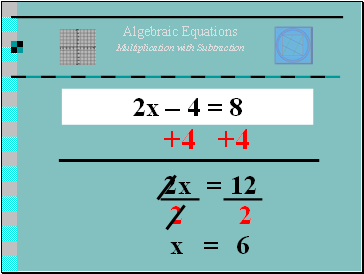Algebraic Equations

## Multiplication with Subtraction

2x – 4 = 8

+4

+4

2x

=

12

2

2

x

=

6

Slide 3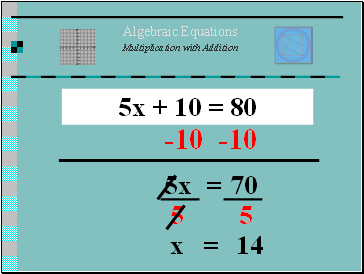Algebraic Equations

5x + 10 = 80

-10

-10

5x

=

70

5

5

x

=

14

Slide 4Algebraic Equations

Multiplication with Subtraction

-3x – 4 = -82

+4

+4

-3x

=

-78

-3

-3

x

=

26

Slide 5Algebraic Equations

2/3x + 2 = 8

-2

-2

x

=

6

x

=

18/2 = 9

(HINT)

Reciprocal

Slide 6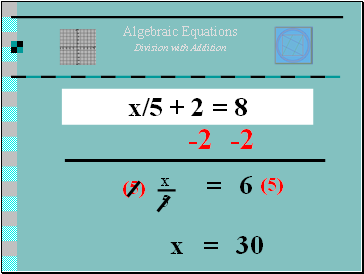Algebraic Equations

x/5 + 2 = 8

-2

-2

=

6

x

=

30

Slide 7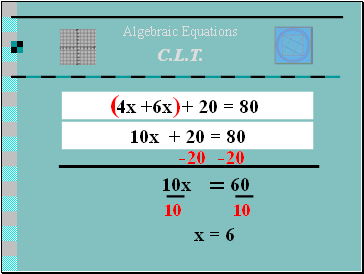Algebraic Equations

## C.L.T.

-20

-20

10x

=

60

10

4x +6x + 20 = 80

10x + 20 = 80

10

x = 6

Slide 8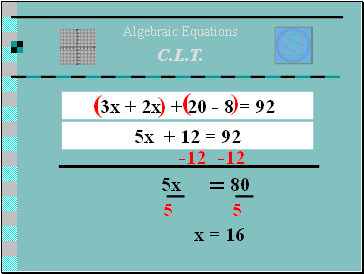Algebraic Equations

C.L.T.

-12

-12

5x

=

80

5

3x + 2x + 20 - 8 = 92

5x + 12 = 92

5

x = 16

Slide 9Algebraic Equations

C.L.T.

-12

-12

2x + 12

=

122

2

6x + 15 = 125 + 4x

6x – 4x + 12 = 122 + 4x – 4x

2

2x = 110

( )

( )

X = 55

Slide 10## Distributive Property

( )

10x – 3x -12 = 4x – 9x + 48

Algebraic Equations

2(5x – 6) – 3x = 4(x + 12) – 9x

10x – 12 - 3x = 4x + 48 – 9x

( )

( )

Slide 1112

12

+12

+12

X = 5

Slide 12THE END

Homework:

Study Review Sheet

Test Tomorrow!!

Use Worksheets & Notes to review and practice for the test.